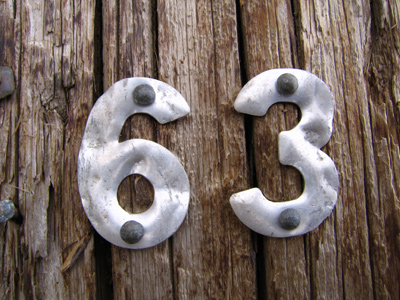13 + 14 + 36 = 63.

This Math quiz is called 'Addition and Subtraction 6' and it has been written by teachers to help you if you are studying the subject at elementary school. Playing educational quizzes is an enjoyable way to learn if you are in the 3rd, 4th or 5th grade - aged 8 to 11.

It costs only \$12.50 per month to play this quiz and over 3,500 others that help you with your school work. You can subscribe on the page at Join Us

Subtraction is taking away a number from another number. Addition and subtraction are important skills: you've got to be able to check that you've been given the right change, haven't you? This quiz will give you a bit of practice in adding and subtracting.

Try to do this stuff in your head - that way next time you are out shopping, you'll know immediately if you've been short-changed or not!

In each of the following questions, what is the answer to the given problem?
1.
47 - 58 + 19 = ?
8
7
6
9
TIP: With numbers less than 100, add the tens first and then add the units. 47 - 58 + 19 = (40 + 20 - 50) + (7 - 8 - 1) = 10 - 2 = 8. Here '19' was replaced by '20 - 1' and the calculation was done using using this
2.
37 - 19 = ?
19
16
18
17
TIP: With numbers less than 100, add the tens first and then add the units. 37 - 19 = (30 - 10) + (7 - 9) = 20 - 2 = 18
3.
0 + 10 + 0 + 28 = ?
48
58
0
38
TIP: With numbers less than 100, add the tens first and then add the units. 0 + 10 + 0 + 28 = (10 + 20) + (8) = 30 + 8 = 38. Ignore zeros!
4.
16 + 17 = ?
35
36
33
37
TIP: With numbers less than 100, add the tens first and then add the units. 16 + 17 = (10 + 10) + (6 + 7) = 20 + 13 = 33
5.
6 + 6 + 6 - 6 = ?
18
24
12
6
TIP: With numbers less than 100, add the tens first and then add the units. (6 + 6 + 6) - 6 = 18 - 6 = 12
6.
19 - 27 + 56 = ?
49
51
48
53
TIP: With numbers less than 100, add the tens first and then add the units. 19 - 27 + 56 = (10 + 50 - 20) + (9 + 6 - 7) = 40 + 8 = 48. You could write '19' as '20 - 1' and do the calculation using this. Always BE ON THE LOOKOUT for useful simplifications
7.
13 + 14 + 36 = ?
63
61
62
65
TIP: With numbers less than 100, add the tens first and then add the units. 13 + 14 + 36 = (10 + 10 + 30) + (3 + 4 + 6) = 50 + 13 = 63. Don't forget: sometimes you will spot an easy addition which will make the calculation easier, e.g. 14 + 36 = 50
8.
25 - 47 + 89 - 5 = ?
61
62
64
63
TIP: With numbers less than 100, add the tens first and then add the units. 25 - 47 + 89 - 5 = (20 + 80 - 40) + (5 + 9 - 7 - 5) = 60 + 2 = 62
9.
27 + 46 + 83 = ?
168
158
165
156
TIP: With numbers less than 100, add the tens first and then add the units. 27 + 46 + 83 = (20 + 40 + 80) + (7 + 6 + 3) = 140 + 16 = 156
10.
3 - 6 + 17 + 28 = ?
44
42
43
46
TIP: With numbers less than 100, add the tens first and then add the units. 3 - 6 + 17 + 28 = (10 + 20) + (7 + 8 + 3) - (6) = 30 + 18 - 6 = 48 - 6 = 42
Author:  Frank Evans